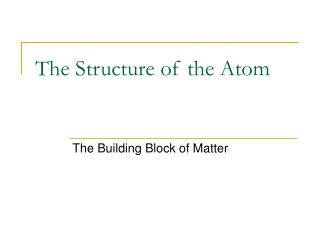DownloadDownload PresentationThe Structure of the Atom

# The Structure of the Atom

Télécharger la présentation## The Structure of the Atom

- - - - - - - - - - - - - - - - - - - - - - - - - - - E N D - - - - - - - - - - - - - - - - - - - - - - - - - - -
##### Presentation Transcript

1. The Structure of the Atom The Building Block of Matter

2. Atoms are the smallest particles of an element that retains the chemical properties of that element • Parts: • Nucleus – small region in the center of the atom. (+ charged protons, = charged neutrons) • Electron Cloud – large region, compared to nucleus, where electrons are found.

3. Size of nucleus vs. electron cloud If an atom was the size of a sports stadium, the nucleus would be the size of a marble! Subatomic Particles: • Protons : + charge, relative mass = 1.007 atomic mass units (amu); round to 1 • Neutrons: = charge, relative mass = 1.009 atomic mass units (amu); round to 1 • Electrons: - charge, relative mass = 0.0005 atomic mass units (amu); round to 0 (not factored in when figuring total mass of an atom)

4. Structure of Subatomic Particles • Subatomic particles composed of fast moving points of energy called quarks Quark Calculations(for protons and neutrons) Each proton is 2 up quarks and 1 down quark 2(2/3) – 1(1/3) = 4/3 – 1/3 = 3/3 or +1 Each neutron is 2 down quarks and 1 up quark 2(-1/3) + 2/3 = 0 Each electron is composed of 2 down quarks 3(-1/3) = -1

5. Isotopes • Atoms with the same number of protons & electrons but a different number of neutrons. • They are the same element, but have different masses. • All isotopes are used to calculate atomic mass (reason why weight is a decimal). • Most elements consist of a mixture of isotopes.

6. Atomic Number • Number of protons in the nucleus (also the number of electrons) • Typically the number on top of the symbol on the Periodic Table. 13 Atomic Number 26.981

7. Mass Number • Number of protons + number of neutrons in an atom. • When solving for number of neutrons: round mass number properly, subtract atomic number from mass number.

8. Element Symbols • Hyphen Notation: Element symbol – Mass # (rounded) Example: Helium  He-4 • Nuclear Symbol: Superscript Mass Number, Subscript Atomic Number, Symbol Example: 4 He 2